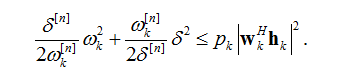# How to deal with {real affine} .* {convex}

Hello everyone,Please help meIn the picture, pk, delta and omega are variables.Left-hand side of inequality, delta[n] and omega[n] are constant,that’s the value of the nth iteration. Right hand side of inequality, wkH and hk are known.These are the two versions of the code that I wrote,In the code,y(a_off(ii)) represents the right-hand side of the above inequality
relax_scaler_delta_ini*pow_p(relax_scaler_omega(a_off(ii)),2)inv_pos(2relax_scaler_omega_ini(a_off(ii)))… + relax_scaler_omega_ini(a_off(ii))*pow_p(relax_scaler_delta(a_off(ii)),2)inv_pos(2relax_scaler_delta_ini) <= y(a_off(ii))；

If someone helped me, I would be very grateful.

at a glance there is a coding problem. you use the same variable (omega,delta) to save the constant from last iteration, and be the variable of current iteration, which is confusing and makes no sense.

Thank you for your reply，but I make a distinction：
In the following code, I use the relax_scaler_delta and relax_scaler_omega from the last iteration as the next relax_scaler_delta_ini and relax_scaler_omega_ini,In other words, I use _ini to make a distinction
relax_scaler_delta_ini = relax_scaler_delta;
relax_scaler_omega_ini = relax_scaler_omega

sorry for the mistake. the error says you multiplied two convex expression, which is not permitted in cvx. please point out the line that threw the error, and format the code in markdown format.

Sorry, do you mean that I should convert the code to this form?

relax_scaler_delta_ini*pow_p(relax_scaler_omega(a_off(ii)),2)inv_pos(2relax_scaler_omega_ini(a_off(ii)))+relax_scaler_omega_ini(a_off(ii))*pow_p(relax_scaler_delta(a_off(ii)),2)inv_pos(2relax_scaler_delta_ini) <= y(a_off(ii))；

This is my error

Why are you using `quad_over_lin` or `inv_pos`? From the description in the first post, it appears the constraint is
`constant_1*omega^2 + constant_2*delta^2 <= p*constant_3`
You should be able to enter that “as is” in CVX, presuming constant_1 and constant_2 evaluate to nonnegative. That makes LHS the sum of scalar convex quadratics.

I use quad_over_lin or inv_pos because I found that you gave this suggestion for the similar question in the forum.
May I ask where specifically I should input “as is” ?Can you give me an example?
Thank you for your kind!relax_scaler_delta_ini = relax_scaler_delta;
relax_scaler_omega_ini = relax_scaler_omega
I seem to have made these two constants nonnegative:
relax_scaler_omega>0;
relax_scaler_delta>0;

`quad_over_lin` is only needed if the numerator has a quadratic in a CVX variable and denominator affine in a CVX variable.

I will leave off the subscripts: The code is something like this:

``````variables omega delta p
(delta_n/(2*omega_n))*omega^2 + (omega_n/(2*delta_n))*delta^2 <= p*abs(w'*h)^2``````
1 Like

Why does my code problem turn into this:

{real affine} ./ {real affine}

My revised code looks like this:

`(relax_scaler_delta_ini/(2*relax_scaler_omega_ini(a_off(ii))))*relax_scaler_omega(a_off(ii))^2+ (relax_scaler_omega_ini(a_off(ii))/ (2*relax_scaler_delta_ini))*relax_scaler_delta^2 <= y(a_off(ii));`

Your code doesn’t show variable declarations. Which symbols are declared as CVX variables is crucial. Perhaps you are declaring some things as variables which actually should be input data. My code only declared 3 variables (what you said were the variables); everything else is input data from the standpoint of CVX.

This is my variable declaration

variable trans_power(K)
variable relax_scaler_omega(K)
variable relax_scaler_delta

You are showing so many scattered pieces and error messages, that I don’t know what goes with what, and maybe you don’t either. Do things systematically. if there is a line where you get an error message, examine each subexpression (print it at command line) to see what is going on. if you use my code template, there should be no CVX expression in any denominator.

1 Like

Is it because I have entered relax_scaler_delta_ini, relax_scaler_omega_ini outside the function

function [trans_power, flag_P] = Generate_trans_power(relax_scaler_delta_ini , relax_scaler_omega_ini)

I don’t know,. You are showing bits and pieces, so I don’t know what’s going on. Follow the advice from my previous post, which might have been entered while you were typing.

Slow down, and do things systematically.

Thank you for reminding,I’ll try it your way

Maybe you will see that some of the things you think and want to be constants (input data), are actually CVX expressions, in which case there is something wrong with your code.

1 Like

Ok, thank you very much

Thank you very much. Problem solved. Thank you very much